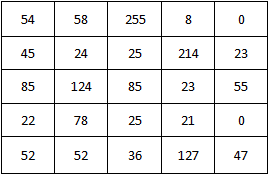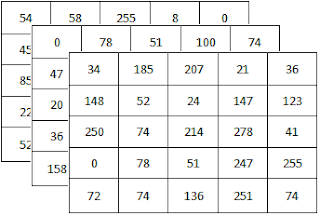### OpenCV C++ API

In this section, I will introduce you some fundamental concepts of OpenCV C++ API. These concepts will help you to understand and write OpenCV C++ example programs in this tutorial more easily.

You will need to include only the opencv2/opencv.hpp header file in your program. That header file will include all the other necessary header files for your application. Therefore you don't need to bother thinking which header files should be included for your program any more.
e.g. -
```#include <opencv2/opencv.hpp>
```

### Namespace

All OpenCV classes and functions are in cv namespace. So, you have to do one of following

• Add the using namespace cv; line just after including header files (I have used this method in all my example programs in this tutorial)
e.g. -
```#include <opencv2/opencv.hpp>
using namespace cv;

int main(int argc, char** argv)
{
Mat image = imread("D:/My OpenCV Website/Eagle.jpg");
return 0;
}

```

• Append the cv:: specifier at the beginning of every OpenCV classes, functions and data structures in your source code
e.g. -
```#include <opencv2/opencv.hpp>

int main(int argc, char** argv)
{
cv::Mat image = cv::imread("D:/My OpenCV Website/Eagle.jpg");
return 0;
}
```

### Data Types of Arrays

Data type of an array defines the number of channels, the number of bits allocated for each element and how the value of an element is represented using those bits. If an array represents an image, each elements of the array are pixels of the image.

Any single channel array should belong to one of following data types.
• CV_8U - 8 bit unsigned integer
• CV_8S - 8 bit signed integer
• CV_16U - 16 bit unsigned integer
• CV_16S - 16 bit signed integer
• CV_32S - 32 bit signed integer
• CV_32F - 32 bit floating point number
• CV_64F - 64 bit float floating point number

Here I have illustrated an array of which the data type is CV_8U. It has one channel. Each element in the channel is 8 bit unsigned integers. Hence each element should have a value range from 0 to 255. A common analogy example for a single channel array is a black and white image. (Pixel value 0 represents black and 255 represents white. Pixel values in between 0 and 255 represents color in between black and white.)Array of which the data type is CV_8U

We can define all of above data types for multi channel arrays. OpenCV supports up to 512 channels. Here I am going to show you how to define CV_8U data type for multi channel arrays.
• CV_8UC1 - Single channel array with 8 bit unsigned integers which is exactly same as CV_8U
• CV_8UC2 - 2 channel array with 8 bit unsigned integers
• CV_8UC3 - 3 channel array with 8 bit unsigned integers
• CV_8UC4 - 4 channel array with 8 bit unsigned integers
• CV_8UC(n) - n channel array with 8 bit unsigned integers (n can be from 1 to 512) )

In the same way, you can derive multi channel data types using any other single-channel data types. (e.g. - CV_16SC3, CV_32FC4, CV_64FC(27), etc)

Example 1:

Here I have illustrated an array of which the data type is CV_8UC3. It has 3 channels. Each element in each channel is 8 bit unsigned integers. Hence each element should have a value range from 0 to 255. Because this is a 3 channel array, array consists of tuples with 3 elements. The first tuple is {54, 0, 34}, second tuple is {58, 78, 185} and so on. A common analogy example for a 3 channel array is a RGB image which consists of red, green and blue channels.Array of which data type is CV_8UC3

Example 2:

Here I have illustrated an array of which the data type is CV_8SC2. It has 2 channels. Each element in each channel is 8 bit signed integers. Hence each element should have a value range from -128 to 127. Because this is a 2 channel array, array consists of tuples with 2 elements. The first tuple is {-85, -127}, second tuple is {25, 23} and so on.Array of which data type is CV_8SC2

Example Usage :

• Mat img1(3, 5, CV_32F ); //Creating a 3 x 5 single-channel array with 32 bit floating point numbers
• Mat img2(23, 53, CV_64FC(5) ); //Creating a 23 x 53 5-channel array with 64 bit floating point numbers
• Mat img3(Size(100, 200), CV_16UC2 ); //100 x 200 2-channel array with 16 bit unsigned integers

Note :

1. After reading this tutorial, it should be obvious to you that the following 3 datatypes are exactly the same.
• CV_8U
• CV_8UC1
• CV_8UC(1)

2. Although CV_32FC4 is a valid data type, CV_32FC5 is not a valid data type. For arrays which consist of more than 4 channels, parentheses should be used to enclose the channel number. e.g. - CV_32FC(5).
3. Some OpenCV functions can handle only a subset of above data types. So, read the documentation before using OpenCV functions.

### Some Insight into Depth and Channels of Images

Any digital image consists of pixels. Every pixel should have some value. The minimum value for a pixel is 0 and it represents black.When the value of the pixel is increased, the intensity of that pixel is also increased. The maximum value which can be assigned for a pixel depends on the number of bits allocated for each pixels. If the number of bits allocated per pixel is 8, then the maximum value of that pixel is 255 (11111111 in binary)

Now what is depth of an image? The image depth means the number of bits allocated for each pixel. If it is 8, each pixel can have a value between 0 and 255. If it is 4, each pixel can have a value between 0 to 15 (1111 in binary).

#### Gray-scale Image

Here is a simple model of an image with a depth of 8 bits. Each small box represents a pixel. So, each box may contain a value between 0 to 255.Gray-scale Image with a depth of 8

Here is some important properties of the above image.
• Image depth is 8 bits.
• Image consists of single channel.
• The height of the image is 4 pixel.
• The width of the image is 5 pixels.
• The resolution of this image is 5 x 4.

This is a gray-scale image (black and white image) because it only consists of a single channel. Therefore this image does not contain any color information. If the value of this pixel is higher, it will be shown more brighter. If the value is low, it will be shown more darker.

#### Color Image

Following image is a simple model of a color image. Color image should consist of at least 3 planes; Red, Green and Blue. Any pixel is a combination of the three values. Any color can be created by combining these 3 basic colors.

Examples -
• (255, 0, 0) represent pure red.
• (0, 255, 0) represent pure green.
• (0, 0, 255) represents pure blue.
• (255, 0, 255) represents pure violate.R, G, B planes of a color image

In the above image, top left pixel is (23, 231, 46). It will be shown as a greenish color because the green value (231) of that pixel is significantly larger than the red (23) and blue (46) value.

Here is some important properties of the above image.
• Image depth is 24 bits. (because each pixel is represented with 8 x 3 bits (8 bits from each channel)
• The image consists of 3 channels.
• The height of the image is 4 pixel.
• The width of the image is 5 pixels.
• The resolution of this image is 5 x 4.

Note -
OpenCV library functions usually read images in BGR format which means blue plane first, green color plane next and the red plane at the end which is exactly the reverse order of the above image.

Next Lesson : Load & Display Image

1.thanks for sharing

2.Really nice!

3.nyc explanation, understood clearly

4.5.In example 1, where you have illustrated an array of which the data type is CV_8UC3 with 3 channels. In 3rd row 4th column of the array at the top the value is 278. Is it normal? (Asking out of curiosity cause I'm a beginner)

6.This is really helpful for beginners. Thanks for sharing.

7.8.This is the code

#include

using namespace cv;

int main(int argc, char** argv)
{
return 0;

}

i run it in visual studio 2019 release x64 but the code runs and it will not open any image.

1.Probably, you gave wrong path. You should check it with if condition. In addition, You should try these //, \\, / instead of \(back slash) in your code. Maybe it works.

9.C++ was always easy for me to understand. Although, when I was studying in the university, many of my peers had difficulties with it.

10.Great and useful.
Thanks

11.This is very helpfull , thank's for sharing

12.Article Source: http://EzineArticles.com/3909264 artificial intelligence course in hyderabad

13.This is my first visit to your blog! We are a team of volunteers and new
initiatives in the same niche. Blog gave us useful information to work. You
have done an amazing job!

artificial intelligence training in Bangalore